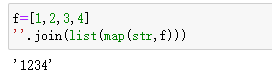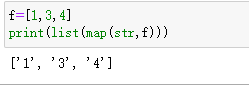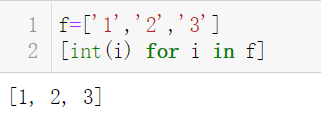• Python列表元素转为数字的方法分析
千次阅读
2021-04-27 03:11:38

本文实例讲述了Python中列表元素转为数字的方法。分享给大家供大家参考，具体如下：

有一个数字字符的列表：

numbers = ['1', '5', '10', '8']

想要把每个元素转换为数字：

numbers = [1, 5, 10, 8]

用一个循环来解决：

new_numbers = [];

for n in numbers:

new_numbers.append(int(n));

numbers = new_numbers;

有没有更简单的语句可以做到呢？

1.

numbers = [ int(x) for x in numbers ]

2. Python2.x,可以使用map函数

numbers = map(int, numbers)

如果是3.x,map返回的是map对象，当然也可以转换为List:

numbers = list(map(int, numbers))

3.还有一种比较复杂点：

for i, v in enumerate(numbers): numbers[i] = int(v)

更多关于Python相关内容感兴趣的读者可查看本站专题：《Python图片操作技巧总结》、《Python数据结构与算法教程》、《Python Socket编程技巧总结》、《Python函数使用技巧总结》、《Python字符串操作技巧汇总》、《Python入门与进阶经典教程》及《Python文件与目录操作技巧汇总》

希望本文所述对大家Python程序设计有所帮助。

您可能感兴趣的文章:python中对数据进行各种排序的方法Python对列表排序的方法实例分析python 把列表转化为字符串的方法Python 对输入的数字进行排序的方法Python将列表中的元素转化为数字并排序的示例

更多相关内容
• 主要介绍了python实现中文日期转换为数字日期，具有很好的参考价值，希望对大家有所帮助。一起跟随小编过来看看吧
• 本文实例讲述了Python列表元素转为数字的方法。分享给大家供大家参考，具体如下： 有一个数字字符的列表： numbers = ['2', '4', '1', '3'] 想要把每个元素转换为数字： numbers = [2, 4, 1, 3] 1. Python2.x，...
• 阿拉伯数字转换为大写数字，主要解决小数点问题，
• ## python中数字列表转化为数字字符串

万次阅读 多人点赞 2020-09-20 11:35:34
1. python数字列表转化字符串或者一串数字 f=[1,2,3,4] num=len(f) m='' #建立空字符串 for i in range(num): x=str(f[i]) m=m+x #利用字符串叠加的方法 print(int(m)) 2.python中国把数字列表转化字符...

# 1. python中数字组成的列表转化为字符串或者一串数字

f=[1,2,3,4]
num=len(f)
m=''  #建立空字符串
for i in range(num):
x=str(f[i])
m=m+x   #利用字符串叠加的方法
print(int(m))
print(m)

显示结果：1234
’1234‘
优化结果：最新方法：用列表表达式：

[str(i) for i in f]  可以一步到位。

# 2.python中把数字组成的列表转化为字符串列表

f=[1,3,4]
new=[]  #建立空列表
for i in f:
q='%d'%i   #格式化占位符，如果是数字转字符就用%s占位即可
new.append(q)
print(new)

显示结果：['1', '3', '4']

以上可以优化如下：
f=[1,3,4]
print(list(map(str,f)))  #一行代码即可实现
显示结果：['1', '3', '4']

如下图：更新使用列表表达式：[str(i) for i in f]  #一步到位

总结：数字列表转化成一串数字，可以用for循环加上字符相加的方法。 数字列表转字符串则可以用占位符格式化，添加新列表即可。

# 3.Python中把字符组成的列表转化为数字

采用join的方法：

num_list=['1','2','3']
str_list = ''.join(num_str)  #把列表中的元素连起来
print(int(str_list))

结果显示：123

# 4.Python中把一串数字字符转化为单个字符列表或者数字列表

用list
a='123'
print(list(a))

显示结果：['1','2','3']

使用map映射函数：

b=['1','2','3']
list(map(int, b)) #把int作用到b中的每一个元素
显示结果：[1,2,3]

更新：列表表达式：[int(i) for i in a.split()]

# 5. Python中把字符串列表转化为数字列表

f=['1','2','3']

最新方法：用列表表达式：

[str(i) for i in f]  可以一步到位。参考文章：

列表之间的数字与字符转化_hu827250322的博客-CSDN博客_列表转换为数字

展开全文字符串 数据结构
• Python具有多种数据处理功能。我们有一个场景，在该场景中，我们给出了一个列表，其中包含一些元素，这些元素...因此，我们设计了一个正则表达式，元组转换为普通字符串，然后应用set函数获得唯一数字。示例import...

Python具有多种数据处理功能。我们有一个场景，在该场景中，我们给出了一个列表，其中包含一些元素，这些元素是一对数字作为元组。在本文中，我们将看到如何从作为元组的列表元素中提取唯一数字。

用重新设置

我们可以使用正则表达式模块及其称为sub的函数。它用于替换匹配正则表达式而不是完全匹配的字符串。因此，我们设计了一个正则表达式，将元组转换为普通字符串，然后应用set函数获得唯一数字。

示例import re

listA = [(21, 3), (13, 4), (15, 7),(8,11)]

# Given list

print("Given list : \n", listA)

temp = re.sub(r'[, ]', '', str(listA))

# Using set

res = [int(i) for i in set(temp)]

# Result

print("List of digits: \n",res)

输出结果

运行上面的代码给我们以下结果-Given list :

[(21, 3), (13, 4), (15, 7), (8, 11)]

List of digits:

[1, 3, 2, 5, 4, 7, 8])

带链套

itertools模块提供了链方法，我们可以使用该方法从列表中获取元素。然后创建一个空集，并继续将元素逐个添加到该集。

示例from itertools import chain

listA = [(21, 3), (13, 4), (15, 7),(8,11)]

# Give

展开全文• 主要介绍了Python简单实现阿拉伯数字和罗马数字的互相转换功能,涉及Python针对字符串与列表的遍历、运算等相关操作技巧,需要的朋友可以参考下
• = 1: return 0 new = ord(old) if 65 答案 5 :(得分：5) [str(ord(c)&31) for c in text] 答案 6 :(得分：2) 这里有一些我用来excel列字母转换数字的东西（所以限制3个字母，但如果你需要更多的话，很容易它...

14 个答案:

答案 0 :(得分：71)

这样的事情：

print [ord(char) - 96 for char in raw_input('Write Text: ').lower()]

修改强>

既然你让我解释我会...虽然已经在[？]的评论中得到了很好的解释。

让我们在更多的一行开始。

input = raw_input('Write Text: ')

input = input.lower()

output = []

for character in input:

number = ord(character) - 96

output.append(number)

print output

这也是一样的，但更具可读性。在您尝试理解我的第一个答案之前，请确保您能够理解这里发生了什么。这里的一切都非常标准，简单的Python。需要注意的一件事是ord函数。 ord代表序数，几乎每种高级语言都有这种功能。它为您提供了任何角色的数字表示的映射。 ord的反函数称为chr。

chr(ord('x')) == 'x' # for any character, not just x.

如果你自己测试，a的序数是97(我上面发布的第三个链接将显示完整的ASCII字符集。)每个小写字母的范围是97-122(26个字符。)所以，如果你只需从任何小写字母的序数中减去96，你将得到它在字母表中的位置，假设你取'a'== 1.所以，序数为'b'== 98，'c'== 99，等等当你减去96，'b'== 2，'c'== 3等等。

我发布的初始解决方案的其余部分只是一些Python技巧，你可以学习称为列表理解。但是，我不会专注于那个，而是专注于学习用任何语言解决问题，其中ord是你的朋友。我希望这会有所帮助。

答案 1 :(得分：11)

您可以使用chr()和ord()来转换字母和整数。

这是一个简单的例子。

>>> chr(97)

'a'

>>> ord('a')

97

答案 2 :(得分：8)

如果您打算大量使用此转换，请考虑计算一次并将结果放入字典中：

>>> import string

>>> di=dict(zip(string.letters,[ord(c)%32 for c in string.letters]))

>>> di['c']

3

优点是字典查找非常快，而不是在每次调用时遍历列表。

>>> for c in sorted(di.keys()):

>>> print "{0}:{1} ".format(c, di[c])

# what you would expect....

答案 3 :(得分：8)

不要太基础，但是这个：

>>> char1 = ['a''b''c''d''e''f''g''h''i''j''k''l'

'm''n''o''p''q''r''s''t''u''v''w''x''y''z']

与此非常不同：

>>> char2 = ['a','b','c','d','e','f','g','h','i','j','k','l',

'm','n','o','p','q','r','s','t','u','v','w','x','y','z']

第一个，没有逗号和你的问题中的内容，是一个带有26个元素字符串的单元素列表。第二个是26个元素列表，每个列表的长度都是一个字符。

如果您打印每个：

>>> print char1, len(char1), len(char1)

['abcdefghijklmnopqrstuvwxyz'] 1 26

>>> print char2, len(char2), len(char2)

['a', 'b', 'c', 'd', 'e', 'f', 'g', 'h', 'i', 'j', 'k', 'l',

'm', 'n', 'o', 'p', 'q','r', 's', 't', 'u', 'v', 'w', 'x', 'y', 'z'] 26 1

很明显，需要额外的一步才能将char1的个别字符转换为iterable。

如果你有sequence个字符'a'到'z'和/或'A'到'Z'，你可以轻松返回list comprehension字符的数字：

>>> [ord(x)%32 for x in char2]

[1, 2, 3, 4, 5, 6, 7, 8, 9, 10, 11, 12, 13, 14, 15, 16,

17, 18, 19, 20, 21, 22, 23, 24, 25, 26]

对于您拥有的数据结构类型，您需要首先访问该字符串：

>>> [ord(x)%32 for x in char1]

[1, 2, 3, 4, 5, 6, 7, 8, 9, 10, 11, 12, 13, 14, 15, 16,

17, 18, 19, 20, 21, 22, 23, 24, 25, 26]

因此，如果您的代码清单与您的问题相同，则可能是您的问题。

合理的替代方案是：[ord(x.lower())-96 for x in char1]

您可以看到，没有逗号的characters=['a''b''c'...]与在['abc...']列表中输入字符串中的所有字符相同。

所以现在尝试：

>>> import string

>>> [ord(x.lower())-96 for x in string.letters]

[1,2,...26, 1,2,3...26] # my ellipses

>>> char3=[string.letters] # one string as element

>>> [ord(x)%32 for x in char3]

>>> [ord(x)%32 for x in [string.letters]]

答案 4 :(得分：6)

这是我过去常用的功能。适用于大写和小写。

def convert_char(old):

if len(old) != 1:

return 0

new = ord(old)

if 65 <= new <= 90:

# Upper case letter

return new - 64

elif 97 <= new <= 122:

# Lower case letter

return new - 96

# Unrecognized character

return 0

答案 5 :(得分：5)

[str(ord(c)&31) for c in text]

答案 6 :(得分：2)

这里有一些我用来将excel列字母转换成数字的东西(所以限制为3个字母，但如果你需要更多的话，很容易将它扩展出来)。可能不是最好的方式，但它适用于我需要它。

def letter_to_number(letters):

letters = letters.lower()

dictionary = {'a':1,'b':2,'c':3,'d':4,'e':5,'f':6,'g':7,'h':8,'i':9,'j':10,'k':11,'l':12,'m':13,'n':14,'o':15,'p':16,'q':17,'r':18,'s':19,'t':20,'u':21,'v':22,'w':23,'x':24,'y':25,'z':26}

strlen = len(letters)

if strlen == 1:

number = dictionary[letters]

elif strlen == 2:

first_letter = letters

first_number = dictionary[first_letter]

second_letter = letters

second_number = dictionary[second_letter]

number = (first_number * 26) + second_number

elif strlen == 3:

first_letter = letters

first_number = dictionary[first_letter]

second_letter = letters

second_number = dictionary[second_letter]

third_letter = letters

third_number = dictionary[third_letter]

number = (first_number * 26 * 26) + (second_number * 26) + third_number

return number

答案 7 :(得分：2)

>>> [str(ord(string.lower(c)) - ord('a') + 1) for c in string.letters]

['1', '2', '3', '4', '5', '6', '7', '8', '9', '10', '11', '12', '13', '14', '15', '16', '17',

'18', '19', '20', '21', '22', '23', '24', '25', '26', '1', '2', '3', '4', '5', '6', '7', '8',

'9', '10', '11', '12', '13', '14', '15', '16', '17', '18', '19', '20', '21', '22', '23', '24',

'25', '26']

答案 8 :(得分：2)

def letter_to_int(letter):

alphabet = list('abcdefghijklmnopqrstuvwxyz')

return alphabet.index(letter) + 1

这里，如果列表包含x，则index(x)函数返回x的位置值。

答案 9 :(得分：1)

如果目标只是转换字母abcd .... xyz和ABCD .... XYZ，我会使用一个函数：

from string import letters

def rank(x, d = dict((letr,n%26+1) for n,letr in enumerate(letters[0:52]))):

return d[x]

我写了[0:52]因为我的Python 2.7版本显示了值

* ABCDEFGHIJKLMNOPQRSTUVWXYZabcdefghijklmnopqrstuvwxyz *ƒŠŒŽšœžŸÀÁÂÃÄÅÆÇÈÉÊËÌÍÎÏÐÑÒÓÔÕÖØÙÚÛÜÝÞßàáâãäåæçèéêëìíîïðñòóôõöøùúûüýþÿ

表示string.letters参数。

因为参数d接收一个值作为默认参数，所以在执行函数定义以生成函数对象时，此值的微积分仅执行一次。

因此，即使该函数被上诉了三千次，也可以在不重新计算该值的情况下使用该函数。

顺便说一下，对于函数的每个上诉，不再使用lower()。在构造默认参数期间，已经处理了大写字母的情况。

使用的一个例子：

word = 'supercalifragilisticexpialidocious'

print ''.join( letter if rank(letter)%3!=0 else '.' for letter in word)

结果：

s.pe..a .... AG ... st..e.p.a..d .....小号

它也可以与map()一起使用：

print map(rank,'ImmunoElectroPhoresis')

结果：

[9,13,13,21,14,15,5,12,5,3,20,18,15,16,8,15,18,5,19,9,19]

答案 10 :(得分：1)

如果您只想将数字映射到一个字母，那么只需执行以下简单的操作：

def letter_to_index(letter):

_alphabet = 'abcdefghijklmnopqrstuvwxyz'

return next((i for i, _letter in enumerate(_alphabet) if _letter == letter), None)

当然如果你想让它从1开始，那么只需添加(i + 1代表我，等等。

答案 11 :(得分：1)

使用此功能。它将一串字母表转换为其等效的数字值：

axis = (^-^) candle^.high candle^.low

答案 12 :(得分：1)

如果您正在查看相反的内容，例如1 = A，2 = B等，则可以使用以下代码。请注意，由于我必须将班级中的部门转换为A，B，C等，因此我最多只能达到2级。

loopvariable = 0

numberofdivisions = 53

while (loopvariable

if(loopvariable<26):

print(chr(65+loopvariable))

loopvariable +=1

if(loopvariable > 26 and loopvariable <53):

print(chr(65)+chr(65+(loopvariable-27)))

答案 13 :(得分：0)

这是我的解决方案，您希望将字母表中的所有字母转换为英文字母中这些字母的位置，并返回这些字符串。

如果您想详细了解它，请告诉我。我使用了以下概念

- 列表理解

- 字典理解

展开全文• 例如：输入[10,2] list = [10,2] strs = [str(num) for l in list] 输出：[‘10’,‘2’]list
• int("".join([str(x)for x in k])) 代码执行的结果如下：list 开发语言
• 本文实例讲述了Python列表元素转为数字的方法。分享给大家供大家参考，具体如下： ... 想要把每个元素转换为数字： 1 numbers =[1, 5, 10, 8] 用一个循环来解决： 1 2 ...
• 我有一个整数列表，我想转换为一个数字，例如：numList = [1, 2, 3]num = magic(numList)print num, type(num)>>> 123, 实现魔术功能的最佳方法是什么？编辑我确实找到了这个，但是似乎必须有一个更好...
• python中的字符数字之间的转换函数int(x [,base ]) x转换为一个整数long(x [,base ]) x转换为一个长整数float(x ) x转换到一个浮点数complex(real [,imag ]) 创建一个复数str(x ) 对象 x 转换为字符串repr(x...
• 今天刷题，碰到了一个需要将数字倒序输出的...在Python里，输入数字转化字符串后，用str()函数，倒序可以用reversed()函数，辅以list()可直接得到数字对应的list。接着可以用该代码： output = int("".join(m...
• 对于使用python进行...带int可以int函数应用于转换为整数的列表的字符串元素。我们必须仔细设计for循环，以遍历每个元素并获得结果，即使单个元素中有多个字符串也是如此。示例listA=[['29','12'],['25'],[...
• 作为Python中数据处理的一部分，有时我们可能需要给定的数字转换为包含该数字列表。在本文中，我们介绍实现这一目标的方法。具有列表理解在下面的方法中，我们str函数应用于给定的数字，然后通过恒等函数...
• 解决方案 Split on commas, then map to integers: map(int, example_string.split(',')) Or use a list comprehension: [int(s) for s in example_string.split(',')] The latter works better on Python 3 if you...
• 主要介绍了python实现range()函数生成的数字存储在一个列表中，具有很好的参考价值，希望对大家有所帮助。一起跟随小编过来看看吧
• 这样的事情： print [ord... 我发布的最初的解决方案的其余部分只是一些Python诡计你可以学习称为列表解析。但是，我不会专注于那么多，因为我会专注于学习解决任何语言的问题，其中ord是你的朋友。我希望这有帮助。
• python中的字符数字之间的转换函数 int(x [,base ]) x转换为一个整数 long(x [,base ]) x转换为一个长整数 float(x ) x转换到一个浮点数 complex(real [,imag ]) 创建一个复数 str(x ) 对象 x 转换...字符串 正则表达式 java
• 1. python数字组成的列表转化字符串或者一串数字f=[1,2,3,4]num=len(f)m='' #建立空字符串for i in range(num):x=str(f[i])m=m+x #利用字符串叠加的方法print(int(m))print(m)显示结果：1234’1234‘优化结果：2...
• 分享给大家供大家参考，具体如下：有一个数字字符的列表：numbers = ['1', '5', '10', '8']想要把每个元素转换为数字：numbers = [1, 5, 10, 8]用一个循环来解决：new_numbers = [];for n in numbers:new_numbers....
• 本文实例讲述了Python列表元素转为数字的方法。分享给大家供大家参考，具体如下： 有一个数字字符的列表： 1 ... numbers = ['1', '5', ... 想要把每个元素转换为数字： 1 numbers = [...
• 在到目前为止，我已经用以下代码创建了一个列表列表：n=4def matrixA(k):A=[]for m in range(0,k):row=[]#A.append([])for n in range(0,k):if (n==(m+1)) or (n==(m-1)):row.append(-deltaX/(de...
• 1.code numb1 = input('输入数字：').split() print(numb1) numb2 = list(map(int, numb1)) print(numb2) 2.result 输入数字：1 2 3 54 ['1', '2', '3', '54'] [1, 2, 3, 54]算法
• python如何把列表转换为集合发布时间：2020-09-21 14:21:24来源：亿速云阅读：146作者：小新小编给大家分享一下python如何把列表转换为集合，相信大部分人都还不怎么了解，因此分享这篇文章给大家参考一下，希望大家......